• C、参考点之间电压 D、参考点之间电阻 5. 焊接时，阴极表面温度很高，阴极中电子运动速度很快，当电子动能大于阴极内部正电荷吸引力时，电子即冲出(　)，产生热发射。 A、负极表面 B、正极表面 C、阴极...
• 入门CFD，主要参考书目《计算流体力学基础及其应用》（John D.Anderson著，吴颂平等译） 实现了第 7.6节激波捕捉 的...如下图所示（图片截取自参考书，下标带0的压力表示总压，亦即初始压力）。激波前的等熵流动...
入门CFD，主要参考书目《计算流体力学基础及其应用》（John D.Anderson 著，吴颂平等 译）

实现了 第 7.6 节 激波捕捉  的代码，采用的是 MacCormack 方法，对守恒型方程求解；关于非守恒型方程，可见亚声速-超声速等熵喷管拟一维流动的CFD解法（附完整代码）。

代码中增加了求解析解的功能。对求解析解过程的理解和思考如下：

1、激波的存在可看做间断点，将激波前后的流动分别看做是两个等熵流动。如下图所示（图片截取自参考书，下标带0的压力表示总压，亦即初始压力）。激波前的等熵流动初始温度为 T0，初始压力为 p0，无初速度；激波后的等熵流动初始温度也为 T0，初始压力为 0.6882p0，无初速度。（ 系数0.6882的计算请往下看，至于为什么可以认为初温相同，欢迎补充 ！）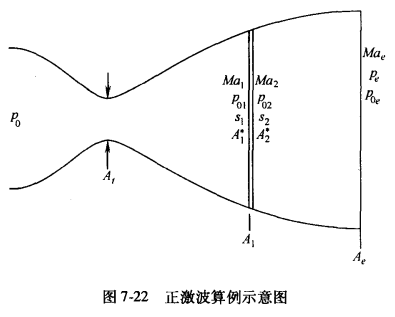2、关联激波前后等熵的流动的要素是质量守恒定律。质量流量由下式计算：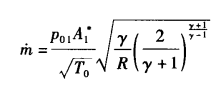式中，A* 代表声速喉道面积。在给定喷管入口条件和喷管形状时，如果流动能够达到声速，则 A* 等于实际喉道面积；如果流动均为亚声速，则 A* 小于实际喉道面积，对应的质量流量也小。由 1 可知质量流量与  成正比。由质量守恒定律可以得到 。

3、结合喷管等熵流动的公式：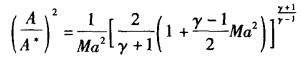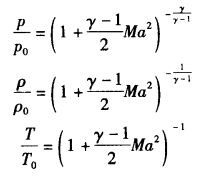可得：

在激波存在时，流动能达到音速， 为实际喉道面积， /  和  /  均可由已知条件确定，据此可以解出  。解出  后，可以计算出 / 。最后，根据下述关系，结合已知条件，即可算出  。

/  是波前马赫数的函数，据此可以解出波前马赫数，从而解出该马赫数对应的截面积，得到激波所在位置。

回顾质量守恒，可以得出  / 的值。最后指出，在同一个问题，统一以激波前的参数为基准确定无量纲物理量的具体数值，因此在计算激波后流动时，需要根据  / 和  /  进行换算。

不足之处，欢迎指正 ！

求解结果：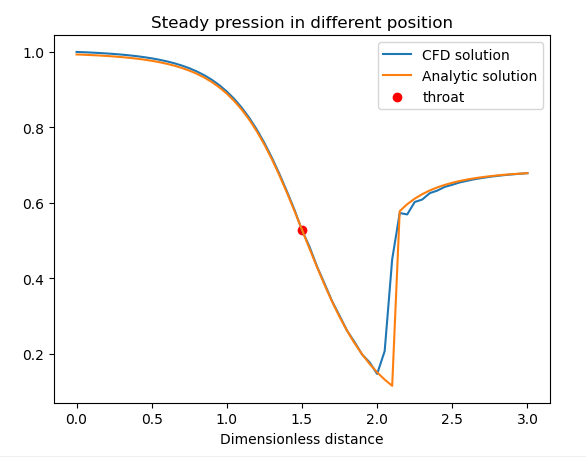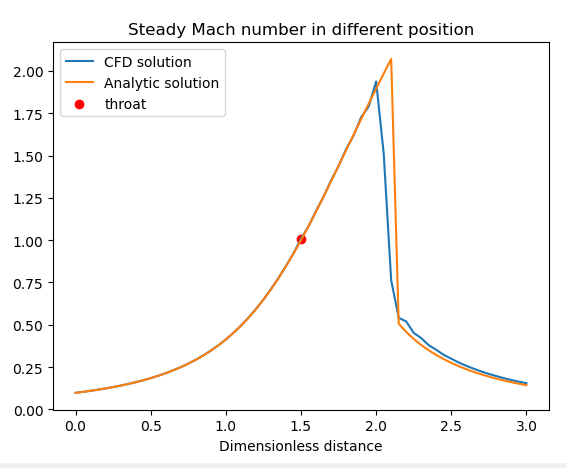求解析解的代码：

#################### 解析解 ########################################################
from scipy.optimize import root

def func(Ma,arg):
return (1.0/1.2*(1.0+0.2*Ma**2.0))**3-arg*Ma #马赫数是面积（位置）的函数

## 激波前
Ma_analytic_1 = []
x_shock = 2.1 ## 激波位置
for x0 in x[np.where(x<=x_shock)]:
A0 = section(x0)
r = root(func, x0=[0,10],args=A0)['x'] #有两个，分别对应喉口两侧
if x0<1.5:
Ma = min(r) # 当 x<1.5 时，Ma<1
else:
Ma = max(r)
Ma_analytic_1.append(Ma)

Ma_analytic_1 = np.array(Ma_analytic_1)
## 激波后
p02_p01 = 0.6882 #激波前后总压比
A1star_a2star = p02_p01 #根据质量守恒，激波前后的声速喉口面积比
Ma_analytic_2 = []
for x0 in x[np.where(x>x_shock)]:
A0 = section(x0)*A1star_a2star ##  激波后喷管无量纲面积, 分母为 A2*
r = root(func, x0=[0,10],args=A0)['x'] #有两个，分别对应喉口两侧
Ma = min(r) # 亚声速
Ma_analytic_2.append(Ma)

Ma_analytic_2 = np.array(Ma_analytic_2)

Ma_analytic = np.hstack([Ma_analytic_1,Ma_analytic_2])

## 激波后的流动等效为 初始温度也是 T0, 初始压力为 p02 = p0*0.6882 的等熵流动
rho_analytic_1 = np.power(1+(gamma-1)/2*Ma_analytic_1**2,-1/(gamma-1))
rho_analytic_2 = np.power(1+(gamma-1)/2*Ma_analytic_2**2,-1/(gamma-1))
rho_analytic = np.hstack([rho_analytic_1, rho_analytic_2*0.6882]) # p = rho*T, rho 跟 p 情况相同

T_analytic_1 = np.power(1+(gamma-1)/2*Ma_analytic_1**2,-1)
T_analytic_2 = np.power(1+(gamma-1)/2*Ma_analytic_2**2,-1)
T_analytic = np.hstack([T_analytic_1, T_analytic_2]) #对于给定的 T0

p_analytic_1 = np.power(1+(gamma-1)/2*Ma_analytic_1**2,-gamma/(gamma-1))
p_analytic_2 = np.power(1+(gamma-1)/2*Ma_analytic_2**2,-gamma/(gamma-1))
p_analytic = np.hstack([p_analytic_1, p_analytic_2*0.6882]) # p02/p0 = 0.6882, 统一换成 p0 的无量纲量

mass_analytic = rho_analytic*A*Ma_analytic*np.sqrt(T_analytic)

####################################################################################

完整代码：

# -*- coding: utf-8 -*-
"""
Created on Wed Aug  5 19:11:15 2020

@author: PANSONG
"""

#### Laval 喷管 拟一维计算 ###############

import numpy as np
import matplotlib.pyplot as plt;plt.close('all')

### 守恒型控制方程，激波捕捉
#%% 建模
#喷管截面形状, 几何建模
def section(x):
return 1+2.2*(x-1.5)**2

# 初始条件
def initial_condition(x):
rho1 = 1 + 0*x[np.where(x<=0.5)]
rho2 = 1.0-0.366*(x[np.where((0.5<x)&(x<=1.5))]-0.5)
rho3 = 0.634-0.702*(x[np.where((1.5<x)&(x<=2.1))]-1.5)
rho4 = 0.5892-0.10228*(x[np.where((2.1<x)&(x<=3.0))]-2.1)

T1 = 1.0 + 0*x[np.where(x<=0.5)]
T2 = 1.0-0.167*(x[np.where((0.5<x)&(x<=1.5))]-0.5)
T3 = 0.833-0.4908*(x[np.where((1.5<x)&(x<=2.1))]-1.5)
T4 = 0.93968-0.0622*(x[np.where((2.1<x)&(x<=3.0))]-2.1)

rho = np.hstack([rho1,rho2,rho3,rho4])
T = np.hstack([T1,T2,T3,T4])

V = 0.59/(rho*section(x))

return rho,T,V

# 边界条件
pe = 0.6784  #出流压力

# 理想气体比热比， 指定材料
gamma = 1.4

## 离散化， 画网格
max_x = 3
dx = 0.05
num_point = int(max_x/dx + 1)
x = np.linspace(0,3,num_point)

mid = np.where(x==1.5) ## 中间点

max_Iteration = 1400

#%% 动态绘图
plt.ion() #开启interactive mode 成功的关键函数
plt.figure()
plt.title('Dimensionless Parameters Evolution')
plt.xlabel('Iteration')

iterations = []

#%%  CFD 计算
#### 初始化，无量纲量
A = section(x)

## 这里给两个初值，一个 0，一个线性，避免迭代过程中 残差判断时 重复判断 i
array0 = np.ones(shape=x.shape)*0.01

rho,T,V = initial_condition(x)
rho_history = np.vstack([array0,rho])
T_history = np.vstack([array0,T])
V_history = np.vstack([array0,V])

## 守恒变量初值计算，这里选择不保存守恒变量
U1 = rho*A
U2 = rho*A*V
U3 = rho*(T/(gamma-1)+gamma/2*V**2)*A
U = np.vstack([U1,U2,U3])

# 辅助变量
J = np.zeros(shape=U[:,2:].shape) # J 维度比 U 和 F 少 2
zero = np.zeros(shape=U.shape) # zero 用于填充偏导数矩阵和人工粘性矩阵，3行1列

C = 0.5 # 柯朗数
Cx = 0.2 # 人工粘性系数
####### MacCormack 方法 ###################################
# 向前，向后差分计算，输入 N 个数据，输出 N-2 个偏导数
def forward_partial(y,dx):
return (y[2:]-y[1:-1])/dx

def backward_partial(y,dx):
return (y[1:-1]-y[:-2])/dx

def calculate_F(U1,U2,U3):
F1 = U2
F2 = U2**2/U1+(gamma-1)/gamma*(U3-gamma/2*U2**2/U1)
F3 = gamma*U2*U3/U1-gamma*(gamma-1)/2*U2**3/U1**2
F = np.vstack([F1,F2,F3])
return F

for i in range(max_Iteration):

# 绘制 喉口处 无量纲温度 和 无量纲密度
iterations.append(i)
if i%20 == 0:
plt.plot(iterations,rho_history[1:,mid],'r-',linewidth=1.0)
plt.plot(iterations,T_history[1:,mid],'b-',linewidth=1.0)
plt.legend(['Density','Temperature'])
plt.pause(0.01)

##########################################
a = np.sqrt(T) #无量纲当地声速
dt = min(C*dx/(V[1:-1]+a[1:-1])) # 计算最大允许时间推进步长

rho_error = max(np.abs((rho_history[i]-rho_history[i-1])/dt))
V_error = max(np.abs((V_history[i]-V_history[i-1])/dt))
T_error = max(np.abs((T_history[i]-T_history[i-1])/dt))
error = max(rho_error,V_error,T_error) ## 取最大残差
if error < 1e-6:
break

###### 预估步 ##################################

F = calculate_F(U[0,:],U[1,:],U[2,:])

# J 维度比 U 和 F 少 2；
J[1,:] = (gamma-1)/gamma/A[1:-1]*(U[2,1:-1]-gamma/2*U[1,1:-1]**2/U[0,1:-1])*forward_partial(A, dx)
# J[1,:] = 1/gamma*rho[1:-1]*T[1:-1]*forward_partial(A, dx)

P_U_t = -(F[:,2:]-F[:,1:-1])/dx + J  # 向量计算

# 人工粘性
p = rho*T
S = np.abs(p[2:]-2*p[1:-1]+p[:-2])/(p[2:]+2*p[1:-1]+p[:-2])*(U[:,2:]-2*U[:,1:-1]+U[:,:-2])
S = Cx*S

U_pred = U + np.column_stack([zero,P_U_t,zero])*dt + np.column_stack([zero,S,zero])

# 完善入口出预估值
U_pred[1,0] = 2*U_pred[1,1]-U_pred[1,2] # U2, 预估步只插值入流边界，出流边界未用在校正步上
U_pred[2,0] = U_pred[0,0]*(1/(gamma-1)+gamma/2*(U_pred[1,0]/U_pred[0,0])**2) # U3

###### 校正步 ##############################################
F_pred = calculate_F(U_pred[0,:],U_pred[1,:],U_pred[2,:])

J[1,:] = (gamma-1)/gamma/A[1:-1]*(U_pred[2,1:-1]-gamma/2*U_pred[1,1:-1]**2/U_pred[0,1:-1])*backward_partial(A, dx)
# J_pred[1,:] = 1/gamma*rho_pred[1:-1]*T_pred[1:-1]*backward_partial(A, dx)

P_U_t_pred = -(F_pred[:,1:-1]-F_pred[:,:-2])/dx + J

P_U_t_av = 0.5*(P_U_t_pred + P_U_t)

#人工粘性
rho_pred = U_pred[0,:]/A
T_pred = (gamma-1)*(U_pred[2,:]/U_pred[0,:]-gamma/2*(U_pred[1,:]/U_pred[0,:])**2)
p_pred = rho_pred*T_pred
S_pred = np.abs(p_pred[2:]-2*p_pred[1:-1]+p_pred[:-2])/(p_pred[2:]+2*p_pred[1:-1]+p_pred[:-2])*(U_pred[:,2:]-2*U_pred[:,1:-1]+U_pred[:,:-2])
S_pred = Cx*S_pred

U = U + np.column_stack([zero,P_U_t_av,zero])*dt + np.column_stack([zero,S_pred,zero])

# 完善出口校正值
U[:,-1] = 2*U[:,-2]-U[:,-3] # 校正步插值出流边界，用在下一次迭代的预估步
VN = U[1,-1]/U[0,-1]
U[2,-1] = pe*A[-1]/(gamma-1)+gamma/2*U[1,-1]*VN #保证出口处的压力边界条件

## 计算原变量
U[1,0] = 2*U[1,1]-U[1,2] # U2，插值入流边界是为了记录history
U[2,0] = U[0,0]*(1/(gamma-1)+gamma/2*(U[1,0]/U[0,0])**2) #T=1

rho = U[0,:]/A
rho_history = np.vstack([rho_history,rho])

V = U[1,:]/U[0,:]
V_history = np.vstack([V_history,V])

T = (gamma-1)*(U[2,:]/U[0,:]-gamma/2*V**2)
T_history = np.vstack([T_history,T])

plt.ioff() #关闭动态绘图

#%% 计算结果可视化
#################### 解析解 ########################################################
from scipy.optimize import root

def func(Ma,arg):
return (1.0/1.2*(1.0+0.2*Ma**2.0))**3-arg*Ma #马赫数是面积（位置）的函数

## 激波前
Ma_analytic_1 = []
x_shock = 2.1 ## 激波位置
for x0 in x[np.where(x<=x_shock)]:
A0 = section(x0)
r = root(func, x0=[0,10],args=A0)['x'] #有两个，分别对应喉口两侧
if x0<1.5:
Ma = min(r) # 当 x<1.5 时，Ma<1
else:
Ma = max(r)
Ma_analytic_1.append(Ma)

Ma_analytic_1 = np.array(Ma_analytic_1)
## 激波后
p02_p01 = 0.6882 #激波前后总压比
A1star_a2star = p02_p01 #根据质量守恒，激波前后的声速喉口面积比
Ma_analytic_2 = []
for x0 in x[np.where(x>x_shock)]:
A0 = section(x0)*A1star_a2star ##  激波后喷管无量纲面积, 分母为 A2*
r = root(func, x0=[0,10],args=A0)['x'] #有两个，分别对应喉口两侧
Ma = min(r) # 亚声速
Ma_analytic_2.append(Ma)

Ma_analytic_2 = np.array(Ma_analytic_2)

Ma_analytic = np.hstack([Ma_analytic_1,Ma_analytic_2])

## 激波后的流动等效为 初始温度也是 T0, 初始压力为 p02 = p0*0.6882 的等熵流动
rho_analytic_1 = np.power(1+(gamma-1)/2*Ma_analytic_1**2,-1/(gamma-1))
rho_analytic_2 = np.power(1+(gamma-1)/2*Ma_analytic_2**2,-1/(gamma-1))
rho_analytic = np.hstack([rho_analytic_1, rho_analytic_2*0.6882]) # p = rho*T, rho 跟 p 情况相同

T_analytic_1 = np.power(1+(gamma-1)/2*Ma_analytic_1**2,-1)
T_analytic_2 = np.power(1+(gamma-1)/2*Ma_analytic_2**2,-1)
T_analytic = np.hstack([T_analytic_1, T_analytic_2]) #对于给定的 T0

p_analytic_1 = np.power(1+(gamma-1)/2*Ma_analytic_1**2,-gamma/(gamma-1))
p_analytic_2 = np.power(1+(gamma-1)/2*Ma_analytic_2**2,-gamma/(gamma-1))
p_analytic = np.hstack([p_analytic_1, p_analytic_2*0.6882]) # p02/p0 = 0.6882, 统一换成 p0 的无量纲量

mass_analytic = rho_analytic*A*Ma_analytic*np.sqrt(T_analytic)

####################################################################################
def plot_results(x,y,x0,y0,title='value'):
plt.figure()
plt.plot(x,y) ## CFD
plt.plot(x,y) ## analytic
plt.scatter(x0,y0,color='red')
plt.xlabel('Dimensionless distance')
plt.title('Steady '+title+' in different position')
plt.legend(['CFD solution','Analytic solution','throat'])

# plot_results([x,x],[rho_history[-1,:],rho_analytic],1.5,rho_history[-1,mid],title='density')
# plot_results([x,x],[T_history[-1,:],T_analytic],1.5,T_history[-1,mid],title='temperature')

p_history = rho_history*T_history
plot_results([x,x],[p_history[-1,:],p_analytic],1.5,p_history[-1,mid],title='pression')

Ma_history = V_history/np.sqrt(T_history)
plot_results([x,x],[Ma_history[-1,:],Ma_analytic],1.5,Ma_history[-1,mid],title='Mach number')

mass_history = rho_history*A*V_history
plot_results([x,x],[mass_history[-1,:],mass_analytic],1.5,mass_history[-1,mid],title='mass flow')
plt.ylim([0.4,0.7])

plt.show()




展开全文python
• ## 一个牛人提供的GIS源码(很好)

千次下载 热门讨论 2010-08-25 22:49:21
并不因为写些东西太累或者太占时间而停止了更新，也许就如同前文说是因为今天喝多了酒(呵呵，玩笑)，也许如同MSN副标题所言：严重的压力和抑郁，强烈的人格分裂和精神分裂，等待崩溃(呵呵，又一个玩笑)。可能...
• 降低业务方的压力ÿ0c;另外如果业务方节点挂掉ÿ0c;网关也能够返回自身的缓存。 限流 限流对于每个业务组件来说ÿ0c;可以说都一个必须的组件ÿ0c;如果限流做不好的话ÿ0c;当请求量突增时ÿ0c;很...
• 所谓“工欲善其事，必先利其器”，好工具确能起到事半工倍作用。我用到最多就两个JConsole 和JProfiler 。JConsole监控系统内存变化情况，如果有内存溢出话...资源JProfiler_6_0_2版本，压缩包里附带了SN。
• 很多系统的数 据相互冗余和重复的，数据的不一致性会给管理工作带来很大的压力。业务和业务之间的相关性也越来越大，例如公司的计费系统和财务系统，财务系统和人事系 统之间都不可避免的有着密切的关系。 为了...
• 发送表Stat=0表示还没有发送，stat=1时候开始发送，stat=2时候对方已经确认收到，这个时候需要查阅RespStat值，如果是0，则发送成功。否则用这个值去错误表查询才能了解发送不成功原因，这个原因是中国移动...
• MTBE产品不合格，一般不是一个原因造成的，而是由很多多个因素共同造成的，而且这几个因素还是相互影响的，例如塔底蒸汽量大，则反灵敏温度也相对上移偏高，塔顶的压力和温度也会增加，间接影响回流量。...
• 更新到最新版本 SDK 平台工具(至少30.0.0)。 将 Android 设备与要运行 adb 电脑连接到同一个局域网，比如连到同一个 WiFi。 在开发者选项中启用无线调试。 在询问要允许在此网络上进行无线调试吗？...
• 因为 defaultProps 中定义了字段默认有含义ÿ0c;因此不会对其进行操作ÿ0c;避免多次定义产生风险。 现在 fit-input 就将 props 透传到了原生 Input 组件上ÿ0c;因此虽然我没有处理各类事件ÿ0c;...
• 第一个部分 leetcode 经典题目解析，包括思路，关键和具体代码实现。 第二部分对于数据结构与算法总结 第三部分 anki 卡片， 将 leetcode 题目按照一定方式记录在 anki 中，方便大家记忆。 第...algorithm cpp interview
• 注：由于Cruise Booking测试由Cruise Booking需求转化而成，所以需求覆盖中默认就覆盖了Cruise Booking需求项 添加对“View Reservations”需求项覆盖 -- Linking Tests to a Requiremnet 将测试...
• 1、测量实验系统电路板中TP91~TP95各测量电压值，并记录。 2、从总体上初步熟悉两部电话单机用空分交换方式进行通话。 3、初步建立程控交换原理系统及电话通信概念。 4、观察并记录一个正常呼叫全过程。 5、...
• 通过采集100万精华文章来执行压力测试，以普通网民联网计算机为参考标准，单台计算机可以在一天内遍历200万网页、采集20万精华文章，100万精华文章仅需5天就可采集完毕。 (4) 正式版与免费版区别在于：正式版...
• 通过采集100万精华文章来执行压力测试，以普通网民联网计算机为参考标准，单台计算机可以在一天内遍历200万网页、采集20万精华文章，100万精华文章仅需5天就可采集完毕。 (4) 正式版与免费版区别在于：正式版...
• 内部包含了atl控件开发以及如何应用，演示了COM包容与集合、自动化、事件和连接、枚举器和集合以及线程管理等等。 ATL实现CDHtmlDialog模板类v1.03 使用了六个类五个模块类演示了atl调用方法 class ...
• 通过采集100万精华文章来执行压力测试，以普通网民联网计算机为参考标准，单台计算机可以在一天内遍历200万网页、采集20万精华文章，100万精华文章仅需5天就可采集完毕。 (4)正式版与免费版区别在于：正式版允许...
• 压缩包内有两个源码包，一个注册机源程序，另一个解密机源程序，一套完整的参考实例。 VC+MapX源码含GPS跟踪演示 VC3D 利用VC编程在界面上实现3D文字 在MFC应用程序中浏览PDF、Word文档文件 vcdialog 自...
• 压缩包内有两个源码包，一个注册机源程序，另一个解密机源程序，一套完整的参考实例。 VC+MapX源码含GPS跟踪演示 VC3D 利用VC编程在界面上实现3D文字 在MFC应用程序中浏览PDF、Word文档文件 vcdialog 自...
• 通过采集100万精华文章来执行压力测试，以普通网民联网计算机为参考标准，单台计算机可以在一天内遍历200万网页、采集20万精华文章，100万精华文章仅需5天就可采集完毕。 (4) 正式版与免费版区别在于：正式版...
• 压缩包内有两个源码包，一个注册机源程序，另一个解密机源程序，一套完整的参考实例。 VC+MapX源码含GPS跟踪演示 VC3D 利用VC编程在界面上实现3D文字 在MFC应用程序中浏览PDF、Word文档文件 vcdialog 自...
• 压缩包内有两个源码包，一个注册机源程序，另一个解密机源程序，一套完整的参考实例。 VC+MapX源码含GPS跟踪演示 VC3D 利用VC编程在界面上实现3D文字 在MFC应用程序中浏览PDF、Word文档文件 vcdialog 自...
• 反映了当时国内单片机应用、开发先进水平，具有重要参考价值。本书第1卷，选编了1989-1991年间177篇文章。 目 录 第一章 单片机系统综合应用技术 1 1.1 且使用 8098单片机体会 2 1.2 单片机冷启动与热...单片机
• 压缩包内有两个源码包，一个注册机源程序，另一个解密机源程序，一套完整的参考实例。 VC+MapX源码含GPS跟踪演示 VC3D 利用VC编程在界面上实现3D文字 在MFC应用程序中浏览PDF、Word文档文件 vcdialog 自...
• 压缩包内有两个源码包，一个注册机源程序，另一个解密机源程序，一套完整的参考实例。 VC+MapX源码含GPS跟踪演示 VC3D 利用VC编程在界面上实现3D文字 在MFC应用程序中浏览PDF、Word文档文件 vcdialog 自...
• HTTP判题机可以通过账号problem_start/problem_end限制其领取任务题目号范围，配合judge.conf中HTTP_DOWNLOAD=0可以让不方便更新判题数据判题机发挥部分功效。 11-30 更新 优化s树莓派4安装脚本，修补漏洞...
• 传统手工方式对图书信息管理已越来越不能适应社会发展需要，尤其随着计算机网络和Internet普及，运用先进信息管理系统对信息进行科学化和网络化管理，已成为图书信息管理系统发展趋势。 系统研发...软件工程